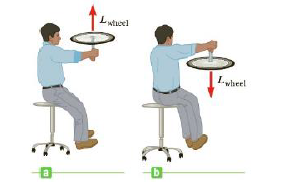Chapter 8, Problem 67P

Chapter
Section
Textbook Problem

A student holds a spinning bicycle wheel while sitting motionless on a stool that is free to rotate about a vertical axis through its center (Fig. P8.67). The wheel spins with an angular speed of 17.5 rad/s and its initial angular momentum is directed up. The wheel’s moment of inertia is 0.150 kg · m2 and the moment of inertia for the student plus stool is 3.00 kg · m2. (a) Find the student’s final angular speed after he turns the wheel over so that it spins at the same speed but with its angular momentum directed down, (b) Will the student’s final angular momentum be directed up or down?Figure P8.67

(a)

To determine
The student’s final angular speed after he turns the wheel over so that it spins at the same speed but with its angular momentum directed down.

Explanation
The initial angular momentum of the wheel is equal to the final angular momenta of wheel and the student and by assuming the angular momentum is positive if directed upward and negative if it is directed downward. So, the conservation of angular momentum of the system is Li=LfIwωw=Iwωw+Isωs . By rearranging this expression, the final angular speed of the student is calculated.

Given info: The moment of inertia of the wheel is 0.150kgm2 , the moment of inertia of the student with stool is 3.00kgm2 , and the initial angular speed of the wheel is 17.5rad/s .

The formula for the final angular speed of the student is,

ωs=2IwωwIs

• Iw is moment of inertia of the wheel.
• ωw is initial angular speed of the wheel.
• Is is moment of inertia plus tool.

Substitute 0.150kgm2 for Iw , 17

(b)

To determine
The direction of final angular momentum of the student.

Still sussing out bartleby?

Check out a sample textbook solution.

See a sample solution

The Solution to Your Study Problems

Bartleby provides explanations to thousands of textbook problems written by our experts, many with advanced degrees!

Get Started